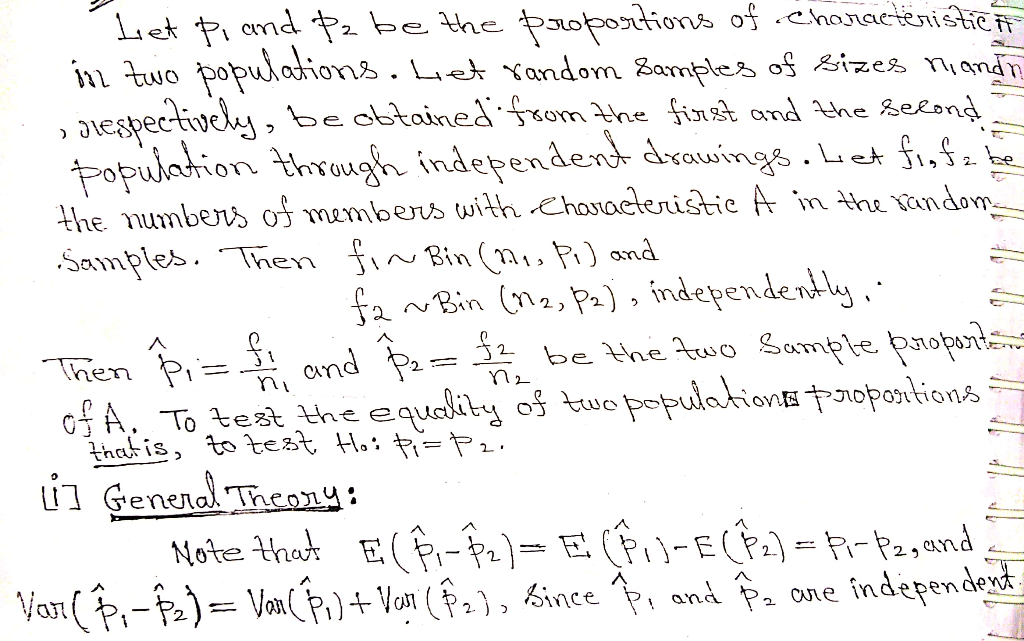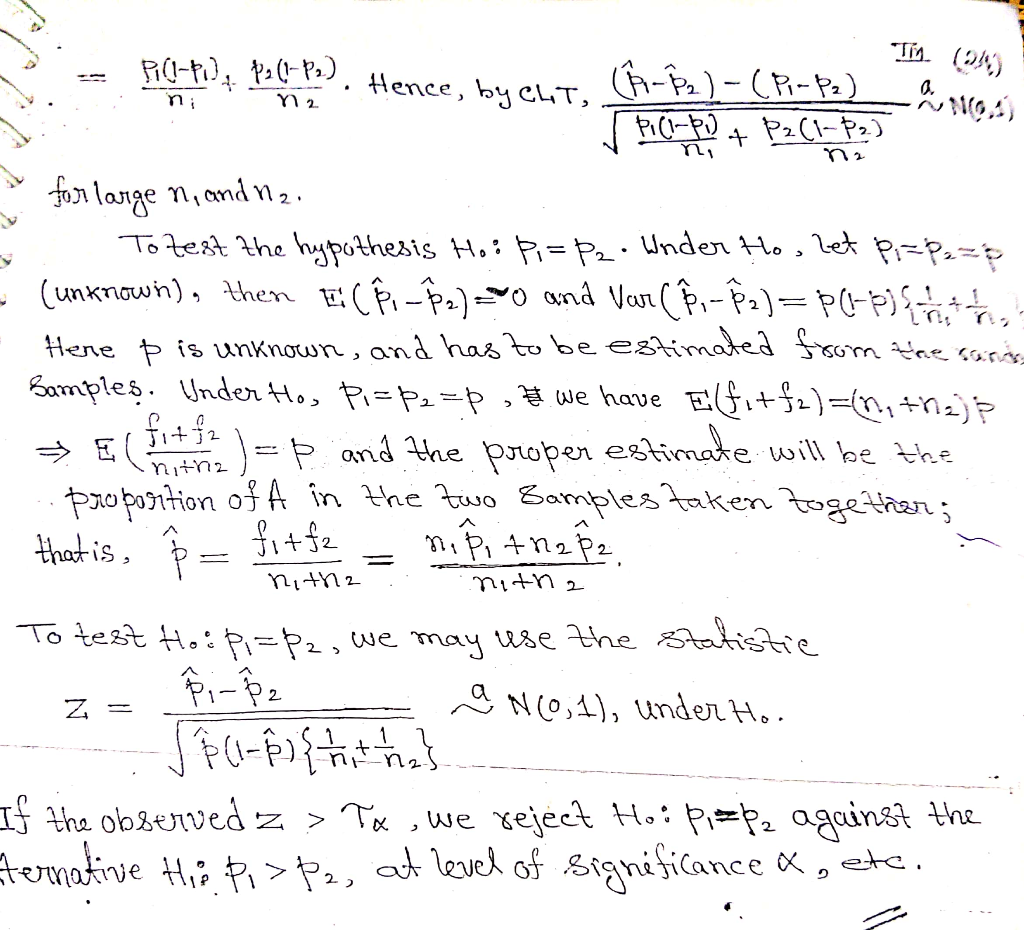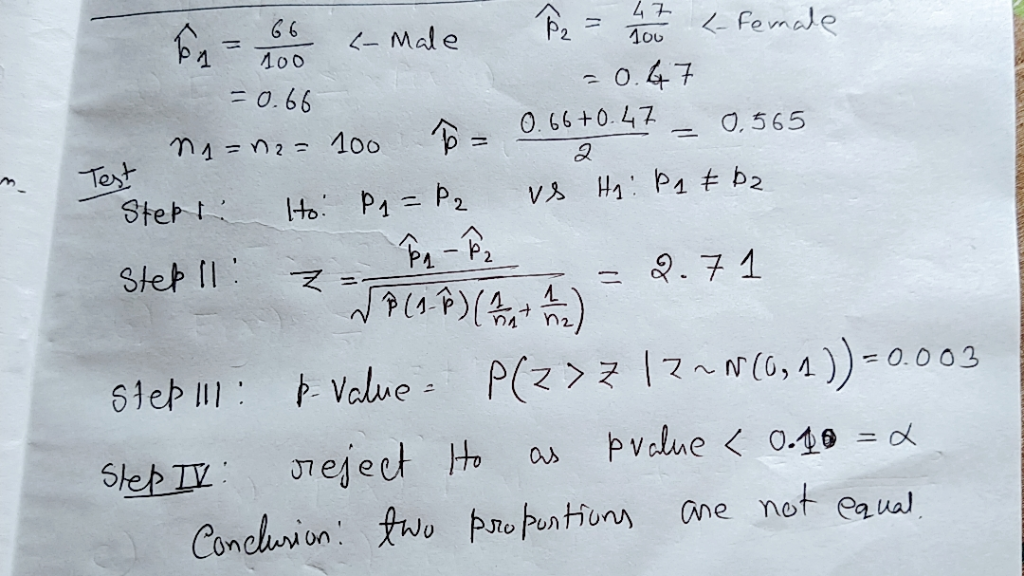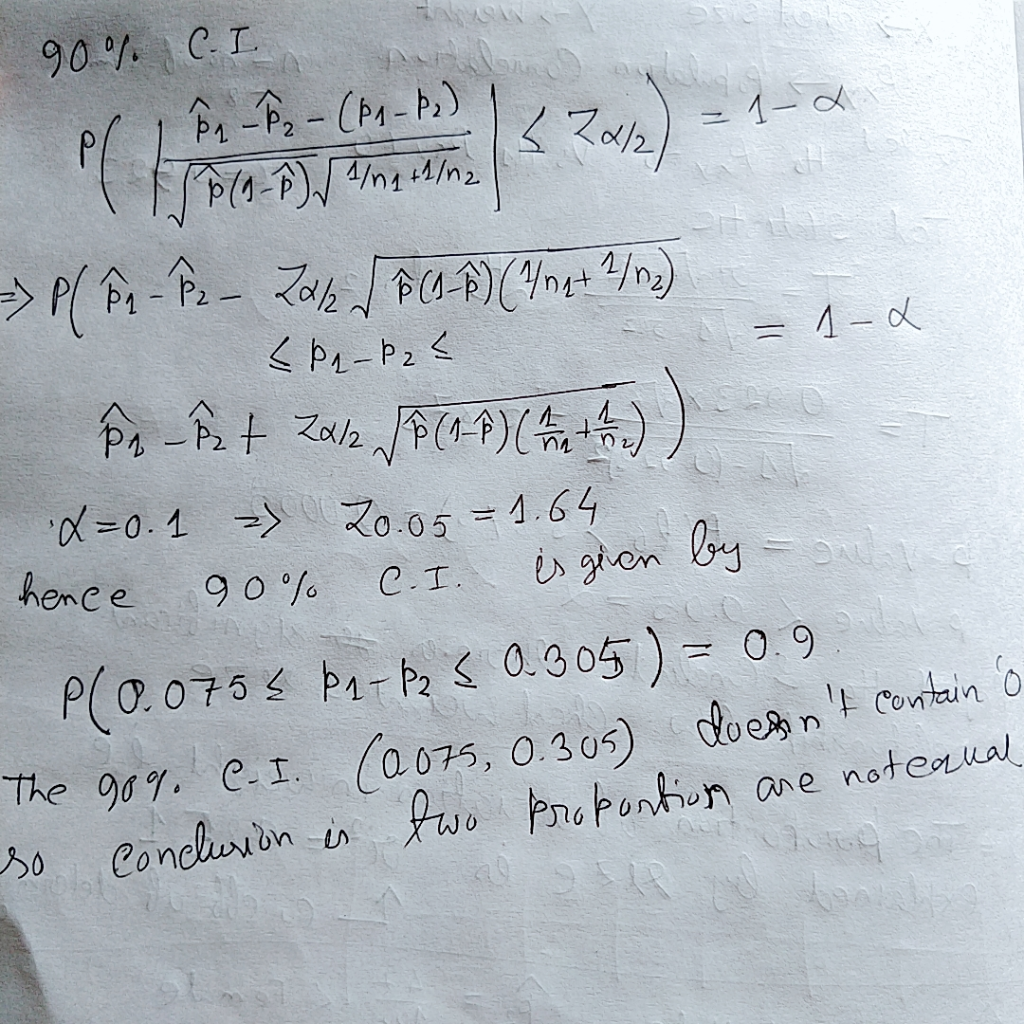##### Question

In: Statistics and Probability

# In a recent survey of county high school students, 100 males and 100 females, 66 of...

In a recent survey of county high school students, 100 males and 100 females, 66 of the male students and 47 of the female students sampled admitted that they consumed alcohol on a regular basis. Find a 90% confidence interval for the difference between the proportion of male and female students that consume alcohol on a regular basis. Can you draw any conclusions from the confidence interval?

For the test of significance questions, clearly indicate each of the formal steps in the test of significance.

Step 1: State the null and alternative hypothesis.
Step 2: Calculate the test statistic.
Step 3: Find the p-value.
Step 4: State your conclusion. (Do not just say “Reject H0” or “Do not reject H0”, state the conclusion in the context of the problem.)

## Solutions

##### Expert Solution## Related Solutions

##### A recent study of high school students shows the percentage of females and males who have...
A recent study of high school students shows the percentage of females and males who have at LEAST one notation in their school record for inappropriate behavior. A simple random sample of high school students was interviewed. The students were asked whether they had a notation for inappropriate behavior in their school record. Of the 100 females, 21 answered yes, as did 145 of the 400 males. Part A: Construct and interpret a 99% confidence interval for the difference in...
##### In a recent survey of high school students, it was found that the amount of time...
In a recent survey of high school students, it was found that the amount of time spent on reading books per week was normally distributed with a mean of 32 minutes. Assume the distribution of weekly reading time follows the normal distribution with a population standard deviation of 2 minutes. Suppose we select a sample of 20 high school students. a. What is the standard error of the mean time? Answer b. What percent of the sample means will be...
##### in a survey of 30 males and 50 females it was found that 15 males and...
in a survey of 30 males and 50 females it was found that 15 males and 20 females exercise regularly. if a person is selected at random what is the probability that: 1.they are male? 2.They are female? 3. They are male who exercise regularly
##### A recent national survey found that high school students watched an average (mean) of 7.2 DVDs...
A recent national survey found that high school students watched an average (mean) of 7.2 DVDs per month with a population standard deviation of 0.90 hour. The distribution of DVDs watched per month follows the normal distribution. A random sample of 35 college students revealed that the mean number of DVDs watched last month was 6.20. At the 0.05 significance level, can we conclude that college students watch fewer DVDs a month than high school students? e. What is the...
##### A recent national survey found that high school students watched an average (mean) of 6.5 movies...
A recent national survey found that high school students watched an average (mean) of 6.5 movies per month with a population standard deviation of 0.6. The distribution of number of movies watched per month follows the normal distribution. A random sample of 33 college students revealed that the mean number of movies watched last month was 5.8. At the 0.05 significance level, can we conclude that college students watch fewer movies a month than high school students? State the null...
##### Question 1 A recent national survey found that high school students watched an average of 6.8...
Question 1 A recent national survey found that high school students watched an average of 6.8 videos per month. A random sample of 36 high school students revealed that the mean number of vidoes watched last month was 6.2. From past experience it is known that the population standard deviation of the number of vidoes watched by high school students is 0.5. At the 0.05 level of signifiance, can we conclude that high school students are watching fewer vidoes? (a)...
##### A recent national survey found that high school students watched an average (mean) of 6.6 DVDs...
A recent national survey found that high school students watched an average (mean) of 6.6 DVDs per month with a population standard deviation of 0.90 hour. The distribution of DVDs watched per month follows the normal distribution. A random sample of 43 college students revealed that the mean number of DVDs watched last month was 6.10. At the 0.05 significance level, can we conclude that college students watch fewer DVDs a month than high school students? d. What is your...
##### A recent national survey found that high school students watched an average (mean) of 6.6 DVDs...
A recent national survey found that high school students watched an average (mean) of 6.6 DVDs per month with a population standard deviation of 0.90 hour. The distribution of DVDs watched per month follows the normal distribution. A random sample of 43 college students revealed that the mean number of DVDs watched last month was 6.10. At the 0.05 significance level, can we conclude that college students watch fewer DVDs a month than high school students? b. State the decision...
##### A recent national survey found that high school students watched an average (mean) of 7.2 movies...
A recent national survey found that high school students watched an average (mean) of 7.2 movies per month with a population standard deviation of 0.7. The distribution of number of movies watched per month follows the normal distribution. A random sample of 47 college students revealed that the mean number of movies watched last month was 6.2. At the 0.05 significance level, can we conclude that college students watch fewer movies a month than high school students? State the null...
##### A recent national survey found that high school students watched an average of 6.8 DVDs per...
A recent national survey found that high school students watched an average of 6.8 DVDs per month with a population standard deviation of 0.5 DVDs. The distribution follows the normal distribution. A random sample of 36 college students revealed that the mean number of DVDs watch last month was 6.2. At the .05 significance level, can we conclude that college students watch fewer DVDs a month than high school students? a. What is the null and alternative hypotheses? b. Is...